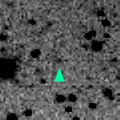# \$B;g6b;3Bh#2WB@1(B

60P/Tsuchinshan 2 (1999)###\$B###\$B50F;MWAG(B

```   The following improved orbital elements by Kenji Muraoka,
are from 89 observations 1965 to 1999, including 9 Planets,
Moon and 5 minor planets perturbations and non-gravitational
effect of style II.  The mean residual is +/- 0.85 arc seconds.

Epoch  =  1999 Mar.  3.0  TT       JDT = 2451240.5
T  =  1999 Mar.  8.18634       +/- 0.00163 (m.e.) TT
Peri. =  203.18608                +/- 0.00085
Node  =  288.20057                +/- 0.00014   (2000.0)
Incl. =    6.71651                +/- 0.00003
q  =    1.7703825              +/- 0.0000022 AU
e  =    0.5063550              +/- 0.0000009
a  =    3.5863478              +/- 0.0000022 AU
n  =    0.14511940             +/- 0.00000014
P  =    6.792                  +/- 0.0000063  years
A1  =   +0.089                  +/- 0.063
A2  =   -0.00327                +/- 0.00022
```

###\$B@1?^(B###\$B8wEYJQ2=(B

```        m1 = 7.5 + 5 log\$B&\$(B + 35 log r(t - 22)
```##### \$B50F;MWAG\$OB<2,7r<#;a\$N7W;;\$K\$h\$k\$b\$N\$G\$9!#(B \$B@1?^\$O(B StellaNavigator Ver.2.0 for Windows (\$B%"%9%H%m%"!<%D(B \$BJTCx(B / \$B%"%9%-!<=PHG6I4)(B) \$B\$G:n@.\$7\$?\$b\$N\$G\$9!#(B \$B8wEY%0%i%U\$O(BComet for Windows\$B\$G:n@.\$7\$?\$b\$N\$G\$9!#(B